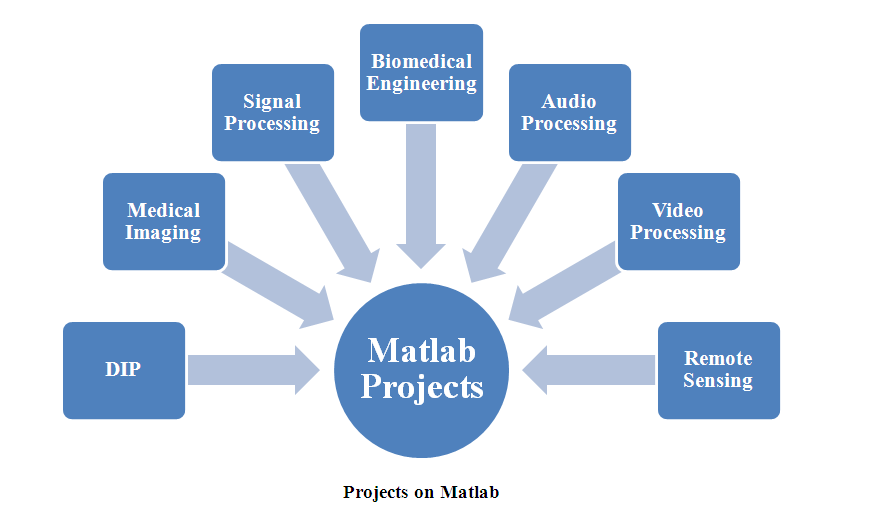# PROJECTS ON MATLAB

Matlab is a software program derived from matrix laboratory and it is named as matlab. It developed with various basic vector and matrix concepts. We support matlab project code to get solution for many problems in algebraic and numerical integration. We develop matlab which is a programming language fourth generation. By matlab, we analyze image properties, feature extraction, edge detection, image segmentation, face recognition applications for B.Tech projects. We support and guide matlab projects for research and academic student based wireless communication network, image processing, and digital signal processing application projects. We ensured an accurate result for data evaluation application and act as a user friendly interface for data evaluation process.

Matlab Basis:

We specially implement matlab to solve mathematical applications. It represented as matrix with row and column. It provides dot products, cross products and inverse matrix. By using a single command function we can add two arrays no need for and while loops optimum graphic output are provided with various color, scale, size and texture are attained by graphical interactive tools.

Variable Definition in Matlab:

Matlab described as simple programming environment and assign variable as numeric values. Various arithmetic operations such as addition, subtraction, multiplication and transpose are also performed by matlab functions and define variables. The common functions utilized by us are:

• Cos – Cosine functions.
• Angle – Angle of complex number.
• Sin – Sine functions.
• Abs – Absolute value for real number.
• Exp – Exponential functions.

These are all represented as radians.Matrix Definition in Matlab:

Matlab considered as basis for matrix and vector algebra. Vector described as arbitrary elements and spaced elements. Arbitrary denoted as V= [1, 2, 3, 4]. Spaced element denoted as V= 1:3:10;

We proposed more than 100+ projects with matlab for image processing projects. We follow the methods to create matrix are:

• Matrix elements are separated by blanks and semicolons.
• Use semicolon (;) to indicate end of statement.
• Matrix elements should enter into square brackets [].

Pre Defined Function To Perform Matrix Operation:

We use following function for operation are:

• Dig (A) to generate sum of Diagonal elements.
• Sum (A) to generate sum of rows and columns.
• Sum (Dig (A)) to generate sum of row wise and column wise diagonals.